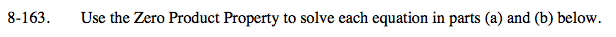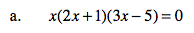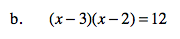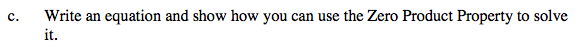Home > A2C > Chapter 8 > Lesson 8.2.4 > Problem8-163

8-163.
1. Use the Zero Product Property to solve each equation in parts (a) and (b) below. Homework Help ✎

1. x(2x + 1)(3x − 5) = 0

2. (x − 3)(x − 2) = 12

3. Write an equation and show how you can use the Zero Product Property to solve it.$\textit{x}=0,\,-\frac{1}{2},\,\frac{5}{3}$Multiply, subtract 12 from both sides, and re-factor before using the Zero Product Property.See parts (a) and (b) for examples.